# E-PolyLearning

 1. Select a suitable figure from the four alternatives that would complete the figure matrix.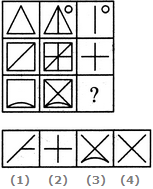a. 1 b. 2 c. 3 d. 4

 2. Select a suitable figure from the four alternatives that would complete the figure matrix.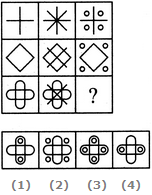a. 1 b. 2 c. 3 d. 4
 3. Select a suitable figure from the four alternatives that would complete the figure matrix.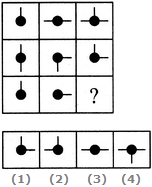a. 1 b. 2 c. 3 d. 4
 4. Select a suitable figure from the four alternatives that would complete the figure matrix.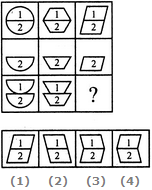a. 1 b. 2 c. 3 d. 4
 5. Select a suitable figure from the four alternatives that would complete the figure matrix.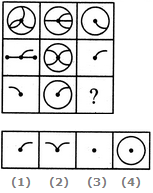a. 1 b. 2 c. 3 d. 4